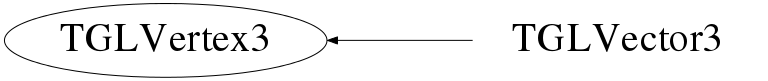# class TGLVertex3

```
TGLVertex3

3 component (x/y/z) vertex class

This is part of collection of simple utility classes for GL only in
TGLUtil.h/cxx. These provide const and non-const accessors Arr() &
CArr() to a GL compatible internal field - so can be used directly
with OpenGL C API calls - which TVector3 etc cannot (easily).
They are not intended to be fully featured just provide minimum
required.

```

## Function Members (Methods)

public:
 virtual ~TGLVertex3() Double_t* Arr() const Double_t* CArr() const static TClass* Class() void Dump() const void Fill(Double_t val) virtual TClass* IsA() const void Maximum(const TGLVertex3& other) void Minimum(const TGLVertex3& other) void Negate() TGLVertex3& operator*=(Double_t f) const TGLVertex3& operator+=(const TGLVector3& val) TGLVertex3 operator-() const const TGLVertex3& operator-=(const TGLVector3& val) TGLVertex3& operator=(const TGLVertex3& rhs) Bool_t operator==(const TGLVertex3& rhs) const Double_t& operator[](Int_t index) const Double_t& operator[](Int_t index) const void Set(const Double_t* xyz) void Set(const TGLVertex3& other) void Set(Double_t x, Double_t y, Double_t z) void Shift(TGLVector3& shift) void Shift(Double_t xDelta, Double_t yDelta, Double_t zDelta) virtual void ShowMembers(TMemberInspector& insp) const virtual void Streamer(TBuffer&) void StreamerNVirtual(TBuffer& ClassDef_StreamerNVirtual_b) TGLVertex3() TGLVertex3(Double_t* v) TGLVertex3(const TGLVertex3& other) TGLVertex3(Double_t x, Double_t y, Double_t z) Double_t X() const Double_t& X() Double_t Y() const Double_t& Y() Double_t Z() const Double_t& Z()
protected:
 Bool_t ValidIndex(UInt_t index) const

## Data Members

protected:
 Double_t fVals

## Class Charts## Function documentation

``` Construct a default (0.0, 0.0, 0.0) vertex
```

``` Construct a vertex with components (x,y,z)
```
TGLVertex3(Double_t* v)
``` Construct a vertex with components (v, v, v)
```
TGLVertex3(const TGLVertex3& other)
``` Construct a vertex from 'other'
```

``` Destroy vertex object
```
void Shift(TGLVector3& shift)
``` Offset a vertex by vector 'shift'
```
void Shift(Double_t xDelta, Double_t yDelta, Double_t zDelta)
``` Offset a vertex by components (xDelta, yDelta, zDelta)
```
void Minimum(const TGLVertex3& other)
void Maximum(const TGLVertex3& other)
void Dump() const
``` Output vertex component values to std::cout
```
void Negate()
void Fill(Double_t val)
void Set(Double_t x, Double_t y, Double_t z)
void Set(const Double_t* xyz)
void Set(const TGLVertex3& other)
Bool_t ValidIndex(UInt_t index) const
``` Fields
```
`{ return (index < 3); }`
Double_t X() const
`{ return fVals; }`
Double_t & X()
`{ return fVals; }`
Double_t Y() const
`{ return fVals; }`
Double_t & Y()
`{ return fVals; }`
Double_t Z() const
`{ return fVals; }`
Double_t & Z()
`{ return fVals; }`
const Double_t * CArr() const
`{ return fVals; }`
Double_t * Arr()
`{ return fVals; }`

`{ MultRight(rhs); return *this; }`
TGLColor& operator=(const TGLVertex3& rhs)